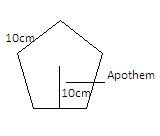QuestionAnswers

# Find the area of a regular pentagon with sides of $10$cm and apothem is $10$cm.A)$251{\text{c}}{{\text{m}}^2}$ B) $250{\text{c}}{{\text{m}}^2}$ C)$252{\text{c}}{{\text{m}}^2}$ D)$253{\text{c}}{{\text{m}}^2}$

Hint: You can use the formula of Area of Pentagon$= \dfrac{5}{2} \times {\text{side of the pentagon}} \times {\text{apothem}}$ .Put the given values and you can get the answer. Here, 5 is the number of sides of pentagon in the formula.

Given, the sides length of regular pentagon=$10$cm and apothem= $10$cm. . A regular pentagon means a pentagon with equal sides and angles.We have to find the area of regular pentagon Now, the formula of area of the pentagon is written as-
$\Rightarrow$ Area of pentagon$= \dfrac{5}{2} \times {\text{side of the pentagon}} \times {\text{apothem}}$.
On putting the given values, we get-
$\Rightarrow {\text{area of pentagon = }}\dfrac{5}{2} \times 10 \times 10$
On multiplying the values we get,
$\Rightarrow {\text{Area of pentagon}} = \dfrac{{500}}{2}$
On division, we have the area of the Pentagon in square cm.
$\Rightarrow {\text{Area of pentagon = 250 c}}{{\text{m}}^2}$
Hence the correct answer is ‘B’.

Note: We can also use the formula ${\text{Area of pentagon = }}\dfrac{{{\text{perimeter}} \times {\text{apothem}}}}{2}$
Where ${\text{Perimeter = number of sides}} \times {\text{side length}}$ . On putting the given value, we get Perimeter=$5 \times 10 = 50$ cm. On putting this value in the formula of area of pentagon, we get-
Area of pentagon=$\dfrac{{50 \times 10}}{2} = \dfrac{{500}}{2} = 250{\text{c}}{{\text{m}}^2}$
In the solution, we simplified the formula by already putting the value of the number of sides of the pentagon. The Pentagon has 5 sides hence it’s called a pentagon.